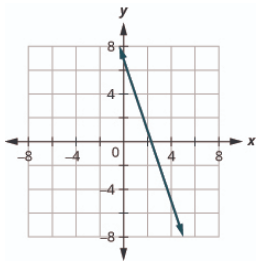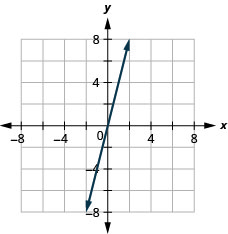# 4.2E: Exercises

•• OpenStax
• OpenStax
$$\newcommand{\vecs}{\overset { \rightharpoonup} {\mathbf{#1}} }$$ $$\newcommand{\vecd}{\overset{-\!-\!\rightharpoonup}{\vphantom{a}\smash {#1}}}$$$$\newcommand{\id}{\mathrm{id}}$$ $$\newcommand{\Span}{\mathrm{span}}$$ $$\newcommand{\kernel}{\mathrm{null}\,}$$ $$\newcommand{\range}{\mathrm{range}\,}$$ $$\newcommand{\RealPart}{\mathrm{Re}}$$ $$\newcommand{\ImaginaryPart}{\mathrm{Im}}$$ $$\newcommand{\Argument}{\mathrm{Arg}}$$ $$\newcommand{\norm}{\| #1 \|}$$ $$\newcommand{\inner}{\langle #1, #2 \rangle}$$ $$\newcommand{\Span}{\mathrm{span}}$$ $$\newcommand{\id}{\mathrm{id}}$$ $$\newcommand{\Span}{\mathrm{span}}$$ $$\newcommand{\kernel}{\mathrm{null}\,}$$ $$\newcommand{\range}{\mathrm{range}\,}$$ $$\newcommand{\RealPart}{\mathrm{Re}}$$ $$\newcommand{\ImaginaryPart}{\mathrm{Im}}$$ $$\newcommand{\Argument}{\mathrm{Arg}}$$ $$\newcommand{\norm}{\| #1 \|}$$ $$\newcommand{\inner}{\langle #1, #2 \rangle}$$ $$\newcommand{\Span}{\mathrm{span}}$$$$\newcommand{\AA}{\unicode[.8,0]{x212B}}$$

## Practice Makes Perfect

Recognize the Relationship Between the Solutions of an Equation and its Graph

In the following exercises, for each ordered pair, decide:

1. Is the ordered pair a solution to the equation?
2. Is the point on the line?

### Exercise $$\PageIndex{1}$$

y=x+2

1. (0,2)
2. (1,2)
3. (−1,1)
4. (−3,−1)1. yes; no
2. no; no
3. yes; yes
4. yes; yes

### Exercise $$\PageIndex{2}$$

y=x−4

1. (0,−4)
2. (3,−1)
3. (2,2)
4. (1,−5)### Exercise $$\PageIndex{3}$$

$$y=\frac{1}{2} x-3$$

1. (0,−3)
2. (2,−2)
3. (−2,−4)
4. (4,1)1. yes; yes
2. yes; yes
3. yes; yes
4. no; no

### Exercise $$\PageIndex{4}$$

$$y=\frac{1}{3} x+2$$

1. (0,2)
2. (3,3)
3. (−3,2)
4. (−6,0)Graph a Linear Equation by Plotting Points

In the following exercises, graph by plotting points.

### Exercise $$\PageIndex{5}$$

$$y=3 x-1$$### Exercise $$\PageIndex{6}$$

$$y=2 x+3$$

### Exercise $$\PageIndex{7}$$

$$y=-2 x+2$$### Exercise $$\PageIndex{8}$$

$$y=-3 x+1$$

### Exercise $$\PageIndex{9}$$

$$y=x+2$$### Exercise $$\PageIndex{10}$$

$$y=x-3$$

### Exercise $$\PageIndex{11}$$

$$y=-x-3$$### Exercise $$\PageIndex{12}$$

$$y=-x-2$$

### Exercise $$\PageIndex{13}$$

$$y=2 x$$### Exercise $$\PageIndex{14}$$

$$y=3 x$$

### Exercise $$\PageIndex{15}$$

$$y=-4 x$$### Exercise $$\PageIndex{16}$$

$$y=-2 x$$

### Exercise $$\PageIndex{17}$$

$$y=\frac{1}{2} x+2$$### Exercise $$\PageIndex{18}$$

$$y=\frac{1}{3} x-1$$

### Exercise $$\PageIndex{19}$$

$$y=\frac{4}{3} x-5$$### Exercise $$\PageIndex{20}$$

$$y=\frac{3}{2} x-3$$

### Exercise $$\PageIndex{21}$$

$$y=-\frac{2}{5} x+1$$### Exercise $$\PageIndex{22}$$

$$y=-\frac{4}{5} x-1$$

### Exercise $$\PageIndex{23}$$

$$y=-\frac{3}{2} x+2$$### Exercise $$\PageIndex{24}$$

$$y=-\frac{5}{3} x+4$$

### Exercise $$\PageIndex{25}$$

$$x+y=6$$### Exercise $$\PageIndex{26}$$

$$x+y=4$$

### Exercise $$\PageIndex{27}$$

$$x+y=-3$$### Exercise $$\PageIndex{28}$$

$$x+y=-2$$

### Exercise $$\PageIndex{29}$$

$$x-y=2$$### Exercise $$\PageIndex{30}$$

$$x-y=1$$

### Exercise $$\PageIndex{31}$$

$$x-y=-1$$### Exercise $$\PageIndex{32}$$

$$x-y=-3$$

### Exercise $$\PageIndex{33}$$

$$3 x+y=7$$### Exercise $$\PageIndex{34}$$

$$5x+y=6$$

### Exercise $$\PageIndex{35}$$

2x+y=−3### Exercise $$\PageIndex{36}$$

$$4x+y=−5$$

### Exercise $$\PageIndex{37}$$

$$\frac{1}{3} x+y=2$$### Exercise $$\PageIndex{38}$$

$$\frac{1}{2} x+y=3$$

### Exercise $$\PageIndex{39}$$

$$\frac{2}{5} x-y=4$$### Exercise $$\PageIndex{40}$$

$$\frac{3}{4} x-y=6$$

### Exercise $$\PageIndex{41}$$

$$2 x+3 y=12$$4x+2y=12

### Exercise $$\PageIndex{43}$$

3x−4y=122x−5y=10

### Exercise $$\PageIndex{45}$$

x−6y=3x−4y=2

### Exercise $$\PageIndex{47}$$

5x+2y=4### Exercise $$\PageIndex{48}$$

3x+5y=5

Graph Vertical and Horizontal Lines

In the following exercises, graph each equation.

### Exercise $$\PageIndex{49}$$

x=4x=3

### Exercise $$\PageIndex{51}$$

x=−2x=−5

### Exercise $$\PageIndex{53}$$

y=3y=1

### Exercise $$\PageIndex{55}$$

y=−5y=−2

### Exercise $$\PageIndex{57}$$

$$x=\frac{7}{3}$$### Exercise $$\PageIndex{58}$$

$$x=\frac{5}{4}$$

### Exercise $$\PageIndex{59}$$

$$y=-\frac{15}{4}$$### Exercise $$\PageIndex{60}$$

$$y=-\frac{5}{3}$$

In the following exercises, graph each pair of equations in the same rectangular coordinate system.

### Exercise $$\PageIndex{61}$$

y=2x and y=2y=5x and y=5

### Exercise $$\PageIndex{63}$$

$$y=-\frac{1}{2} x$$ and $$y=-\frac{1}{2}$$### Exercise $$\PageIndex{64}$$

$$y=-\frac{1}{3} x$$ and $$y=-\frac{1}{3}$$

## Mixed Practice

In the following exercises, graph each equation.

### Exercise $$\PageIndex{65}$$

y=4xy=2x

### Exercise $$\PageIndex{67}$$

$$y=-\frac{1}{2} x+3$$### Exercise $$\PageIndex{68}$$

$$y=\frac{1}{4} x-2$$

### Exercise $$\PageIndex{69}$$

y=−xy=x

### Exercise $$\PageIndex{71}$$

x−y=3x+y=−5

### Exercise $$\PageIndex{73}$$

4x+y=22x+y=6

### Exercise $$\PageIndex{75}$$

y=−1y=5

### Exercise $$\PageIndex{77}$$

2x+6y=125x+2y=10

### Exercise $$\PageIndex{79}$$

x=3x=−4

## Everyday Math

### Exercise $$\PageIndex{81}$$

Motor home cost. The Robinsons rented a motor home for one week to go on vacation. It cost them $594 plus$0.32 per mile to rent the motor home, so the linear equation y=594+0.32x gives the cost, yy, for driving xx miles. Calculate the rental cost for driving 400, 800, and 1200 miles, and then graph the line.

## Writing Exercises

### Exercise $$\PageIndex{83}$$

Explain how you would choose three $$x$$ - values to make a table to graph the line $$y=\frac{1}{5} x-2$$

### Exercise $$\PageIndex{84}$$

What is the difference between the equations of a vertical and a horizontal line?

## Self Check

ⓐ After completing the exercises, use this checklist to evaluate your mastery of the objectives of this section.ⓑ After reviewing this checklist, what will you do to become confident for all goals?

This page titled 4.2E: Exercises is shared under a not declared license and was authored, remixed, and/or curated by OpenStax.